Like   Tweet
 /* styles */ May 13, 2020 Dear Readers, This is the sixth installment of “Expand Your Horizons While Sheltering at Home” from the Dayton Literary Peace Prize. We are dedicated to the belief that reading fine literature is part of the process in making peace a reality. When we read literary works that show us the truth of people’s lives, we are mind-melding with the best thinkers of our time—the fictional characters and real-life people who populate the 2014 finalists bring us to a new understanding and the world becomes less abstract, more familiar. DLPP writers are not naïve, they are realists who present a world with all its flaws, but they write because they have to tell these stories in the hope people will read and know and understand. In 2014, the stories were often prompted by war: war in Chechnya, Spain, France, the Caribbean, Israel and Palestine, Iraq, and the U.S. At home, we hear of the deaths of five young black men, and the endless raging wars against Native Americans, and the shattered lives of soldiers returning from war. These writers also tell the stories of people who work against war. We read of doctors working to save a young child from Russian soldiers, of conscientious objectors in Oregon, the results of war on Nova Scotia, and Muslim moderates working against fanatics in 26 countries throughout the world. Again and again, these authors force us to confront truth. I would argue that our greatest hope lies in those who remain optimistic in an imperfect world. These writers are those rugged optimists and we are grateful to them for spending years dedicated to stories that bring us closer to peace by understanding the horrible consequences of war. Stay safe,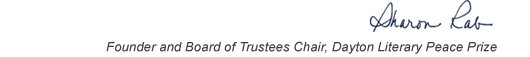table div table+table+table+table div table{width:100%;padding:0}table div table+table+table+table div table img{width:96.23%;padding:0;float:none}table div table+table+table+table div table td{width:100%;padding:0 1.88% 18px}/* styles */This list includes the winners and runners-up for 2014 and we have rich background information on each one on our website.

▪ Under Past Winners. scroll down to the year and click on it.
▪ You will have the option of clicking on a winner, a runner-up, or the finalists.
▪ If you click on the photo of winners and runners-up, you will find the author’s photo and bio, a selection from the book, the judges’ citation, the writer’s reflection on literature and peace, and the option of watching the introduction and acceptance speech.
 ▪ Under Past Winners. scroll down to the year and click on it.
 ▪ You will have the option of clicking on a winner, a runner-up, or the finalists.
 ▪ If you click on the photo of winners and runners-up, you will find the author’s photo and bio, a selection from the book, the judges’ citation, the writer’s reflection on literature and peace, and the option of watching the introduction and acceptance speech.

On the bottom right of the home page, you can click on COREScholar, which is built by the Chair of our Curriculum Committee Carol Loranger, Associate Dean of the College of Liberal Arts at Wright State, and maintained for us by Wright State University. There you can find interviews, TED Talks, articles, other books, films, etc. by and about each of our winning and runner-up authors. It is a great resource for students, book clubs, and readers who would like to explore an author in depth.

 table div table+table+table+table+table+table div table{width:100%;padding:0}table div table+table+table+table+table+table div table img{width:96.23%;padding:0;float:none}table div table+table+table+table+table+table div table td{width:100%;padding:0 1.88% 18px}/* styles *//* styles */ “Read global; buy local.” — Marlon James, DLPP 2009 Fiction Winner for The Book of Night Women
 table div table+table+table+table+table+table+table+table div table{width:100%;padding:0}table div table+table+table+table+table+table+table+table div table img{width:96.23%;padding:0;float:none}table div table+table+table+table+table+table+table+table div table td{width:100%;padding:0 1.88% 18px}/* styles */## 2014 Finalists - Fiction

 table div table+table+table+table+table+table+table+table+table+table div table{width:100%;padding:0}table div table+table+table+table+table+table+table+table+table+table div table img{width:96.23%;padding:0;float:none}table div table+table+table+table+table+table+table+table+table+table div table td{width:100%;padding:0 1.88% 18px}/* styles */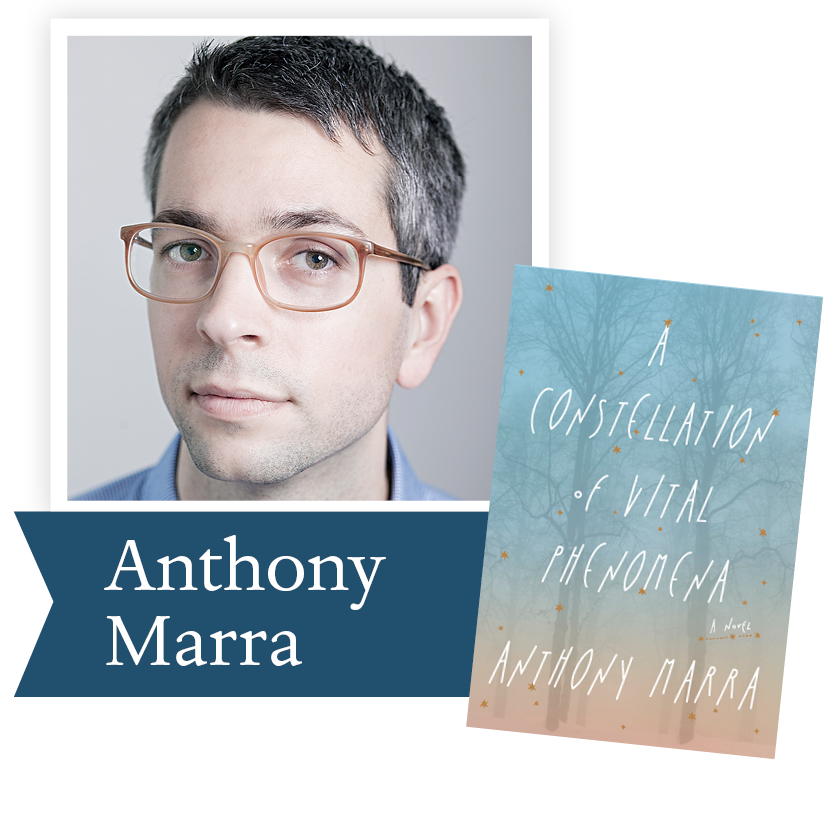A Constellation of Vital Phenomena
by Anthony Marra (Crown Publishing
Group): Two doctors in rural Chechnya risk
everything to save the life of a child hunted
by Russian soldiers in this majestic debut
about love, loss, and the unexpected ties
that bind us together.

 table div table+table+table+table+table+table+table+table+table+table+table+table div table{width:100%;padding:0}table div table+table+table+table+table+table+table+table+table+table+table+table div table img{width:96.23%;padding:0;float:none}table div table+table+table+table+table+table+table+table+table+table+table+table div table td{width:100%;padding:0 1.88% 18px}/* styles */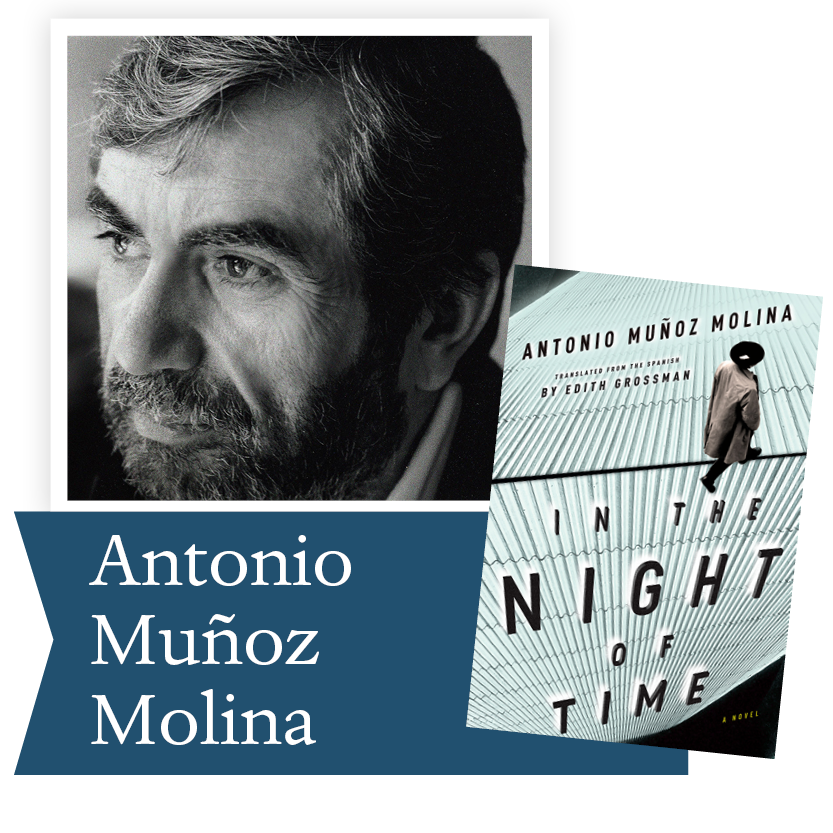In the Night of Time by Antonio Muñoz
Molina (Houghton, Mifflin, Harcourt):
This sweeping, grand novel set against the
tumultuous events that led to the Spanish
Civil War offers an indelible portrait of a
shattered society.

 table div table+table+table+table+table+table+table+table+table+table+table+table+table+table div table{width:100%;padding:0}table div table+table+table+table+table+table+table+table+table+table+table+table+table+table div table img{width:96.23%;padding:0;float:none}table div table+table+table+table+table+table+table+table+table+table+table+table+table+table div table td{width:100%;padding:0 1.88% 18px}/* styles */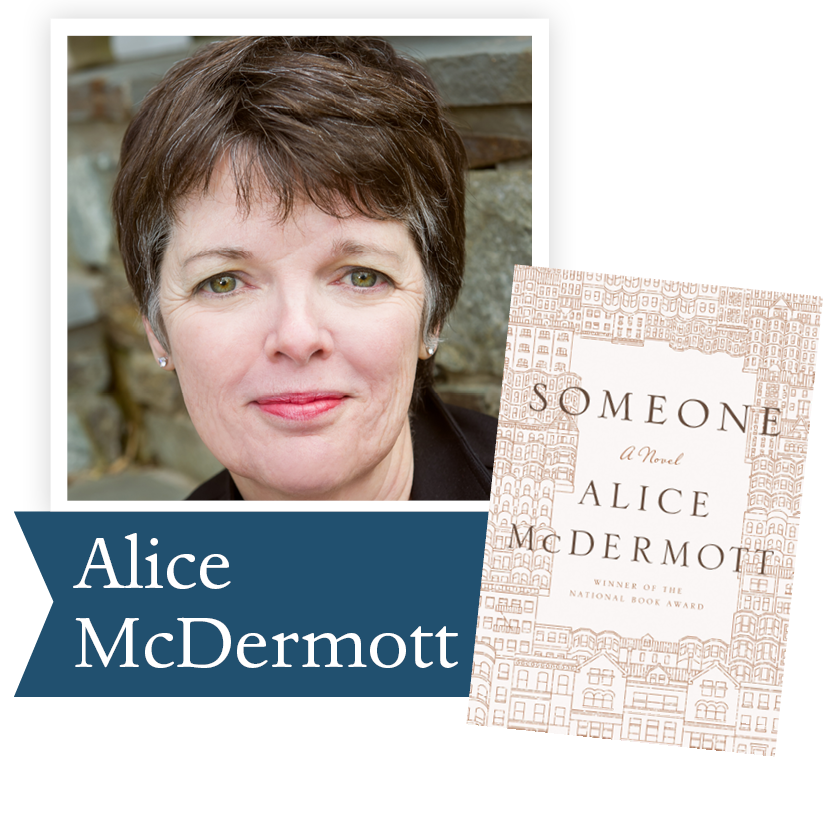Someone by Alice McDermott
(Farrar, Straus and Giroux): In this
delicate narrative about the life of an
ordinary woman, McDermott uses
universal experiences—sharp pains and
unexpected joys, bursts of clarity and
moments of confusion—to deftly arouse
deep compassion for the lives unfolding
all around us.

 table div table+table+table+table+table+table+table+table+table+table+table+table+table+table+table+table div table{width:100%;padding:0}table div table+table+table+table+table+table+table+table+table+table+table+table+table+table+table+table div table img{width:96.23%;padding:0;float:none}table div table+table+table+table+table+table+table+table+table+table+table+table+table+table+table+table div table td{width:100%;padding:0 1.88% 18px}/* styles */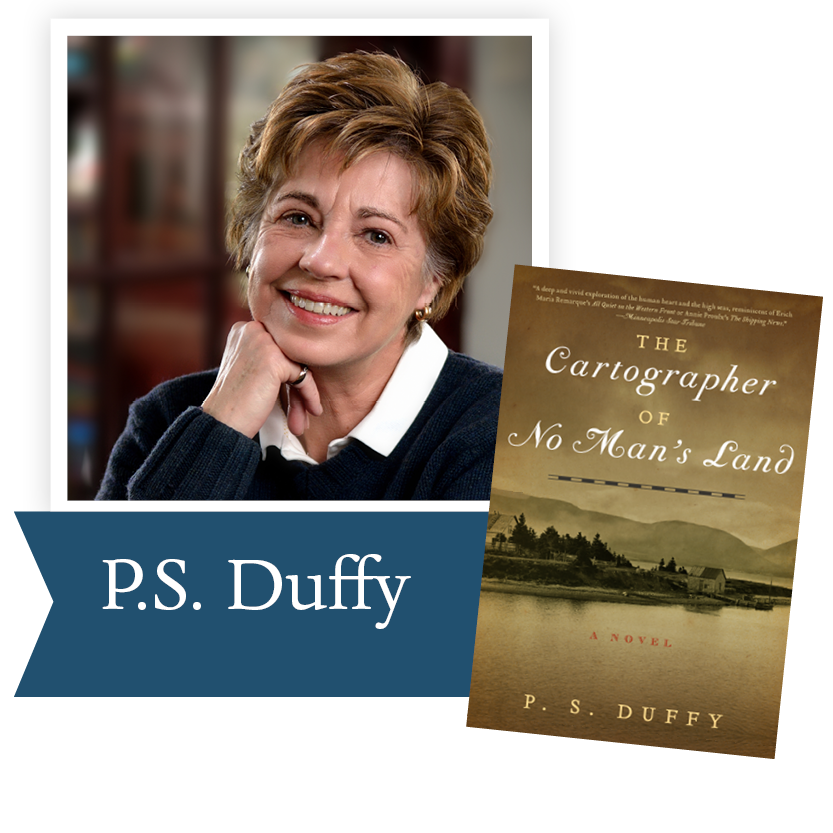The Cartographer of No Man’s Land
by P.S. Duffy (W.W. Norton & Company):
This haunting meditation on family,
friendship, and sacrifice charts a deeply
felt course from the Nova Scotia coastline
to the French trenches during World War I,
bridging the distance between past and
present, duty and honor, obligation
and love.

 table div table+table+table+table+table+table+table+table+table+table+table+table+table+table+table+table+table+table div table{width:100%;padding:0}table div table+table+table+table+table+table+table+table+table+table+table+table+table+table+table+table+table+table div table img{width:96.23%;padding:0;float:none}table div table+table+table+table+table+table+table+table+table+table+table+table+table+table+table+table+table+table div table td{width:100%;padding:0 1.88% 18px}/* styles */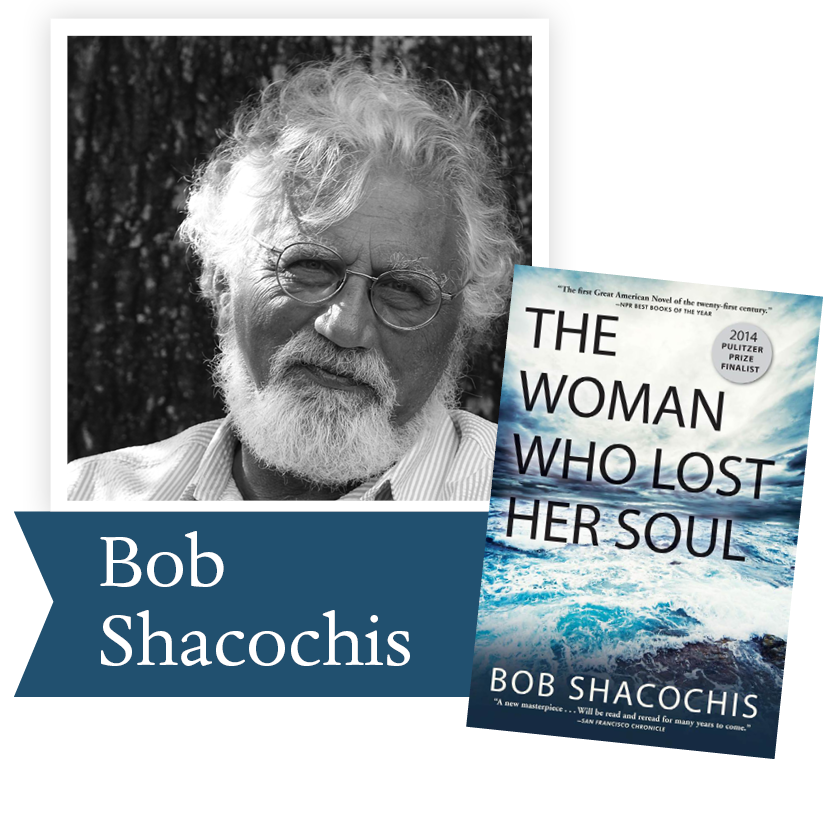The Woman Who Lost Her Soul
by Bob Shacochis (Grove Atlantic):
Renowned for his revelatory visions of the
Caribbean, Shacochis sets his magnum opus in four countries over a span of fifty years and multiple wars, creating an intricate portrait of the catastrophic events that led up to the war on terror and the U.S.
as it is today.

 table div table+table+table+table+table+table+table+table+table+table+table+table+table+table+table+table+table+table+table+table div table{width:100%;padding:0}table div table+table+table+table+table+table+table+table+table+table+table+table+table+table+table+table+table+table+table+table div table img{width:96.23%;padding:0;float:none}table div table+table+table+table+table+table+table+table+table+table+table+table+table+table+table+table+table+table+table+table div table td{width:100%;padding:0 1.88% 18px}/* styles */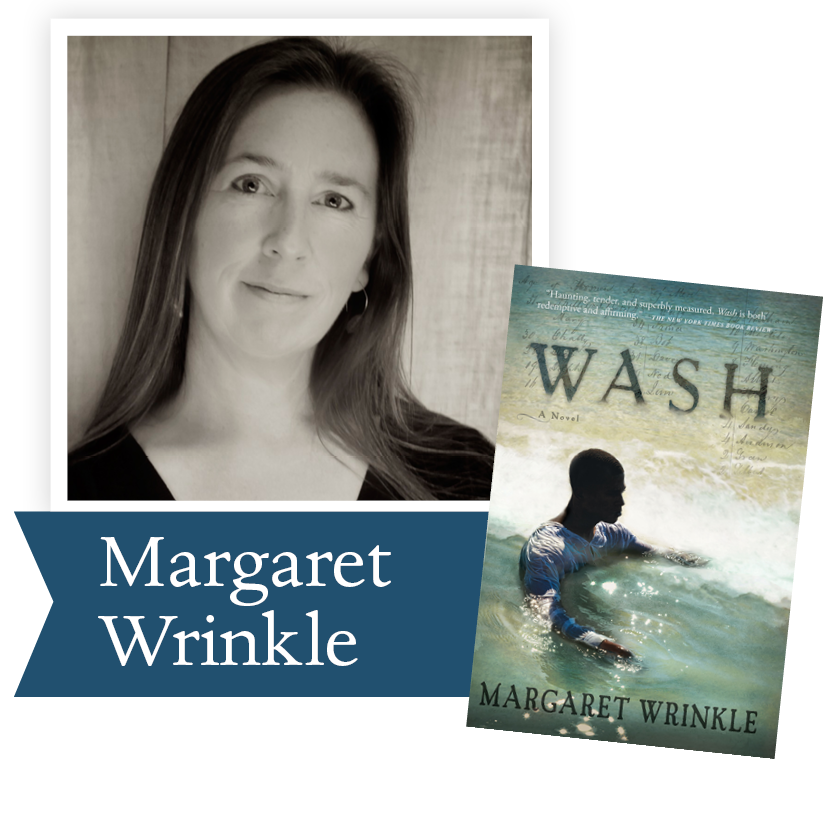Wash by Margaret Wrinkle
(Grove Atlantic): Through the character
of Wash, a first-generation slave, this
haunting first novel explores the oftenburied
history of slave breeding in the early
nineteenth century, offering fresh insights
into our continuing racial dilemmas.

 table div table+table+table+table+table+table+table+table+table+table+table+table+table+table+table+table+table+table+table+table+table+table div table{width:100%;padding:0}table div table+table+table+table+table+table+table+table+table+table+table+table+table+table+table+table+table+table+table+table+table+table div table img{width:96.23%;padding:0;float:none}table div table+table+table+table+table+table+table+table+table+table+table+table+table+table+table+table+table+table+table+table+table+table div table td{width:100%;padding:0 1.88% 18px}/* styles */## 2014 Finalists - Nonfiction

 table div table+table+table+table+table+table+table+table+table+table+table+table+table+table+table+table+table+table+table+table+table+table+table+table div table{width:100%;padding:0}table div table+table+table+table+table+table+table+table+table+table+table+table+table+table+table+table+table+table+table+table+table+table+table+table div table img{width:96.23%;padding:0;float:none}table div table+table+table+table+table+table+table+table+table+table+table+table+table+table+table+table+table+table+table+table+table+table+table+table div table td{width:100%;padding:0 1.88% 18px}/* styles */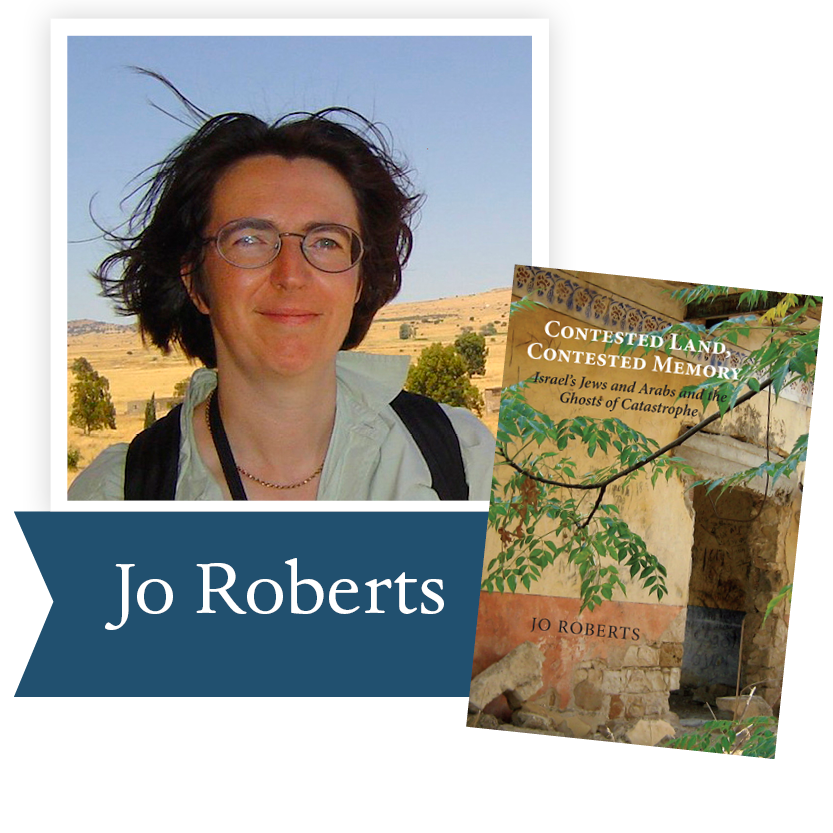Contested Land, Contested Memory: Israel’s Jews and Arabs and the Ghosts of Catastrophe by Jo Roberts
(Dundurn Press, Toronto): Drawing on extensive original interview material, Canadian journalist Jo Roberts vividly examines how their tangled histories of suffering inform Jewish and Palestinian-Israeli lives today, and frame the possibilities for peace in Israel.

 table div table+table+table+table+table+table+table+table+table+table+table+table+table+table+table+table+table+table+table+table+table+table+table+table+table+table div table{width:100%;padding:0}table div table+table+table+table+table+table+table+table+table+table+table+table+table+table+table+table+table+table+table+table+table+table+table+table+table+table div table img{width:96.23%;padding:0;float:none}table div table+table+table+table+table+table+table+table+table+table+table+table+table+table+table+table+table+table+table+table+table+table+table+table+table+table div table td{width:100%;padding:0 1.88% 18px}/* styles */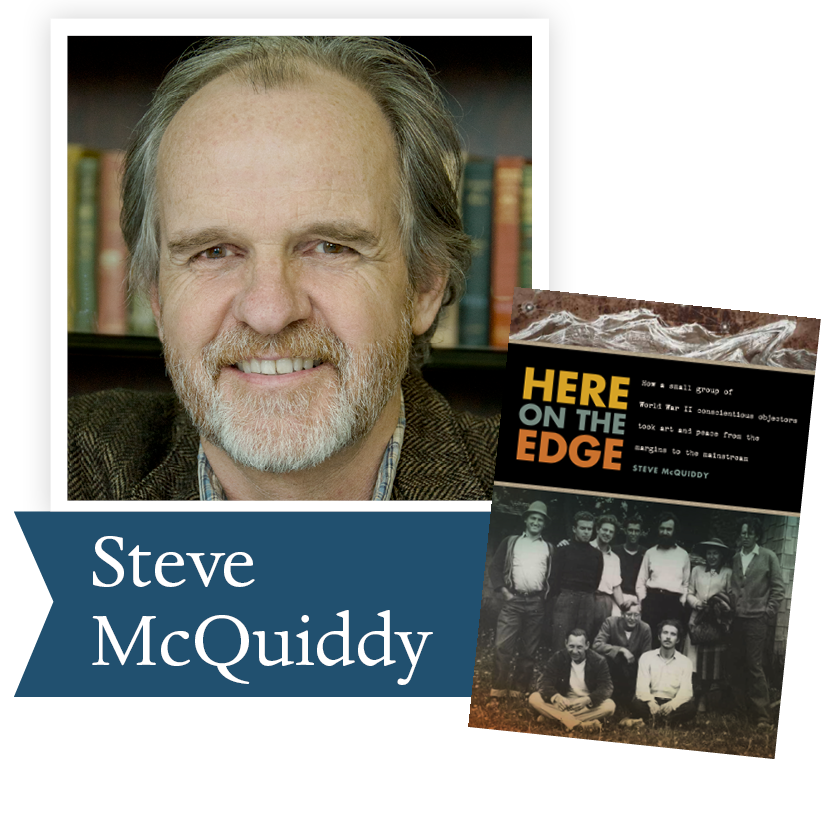Here on the Edge: How a Small Group of WWII Conscientious Objectors Took Art and Peace from the Margins to the Mainstream by Steve McQuiddy (Oregon State University Press): Packed with original
research and more than eighty photos, this definitive history tells the story of the artists at an Oregon camp for World War II conscientious objectors, and how they paved the way for the social and cultural
revolutions of the 1960s.

 table div table+table+table+table+table+table+table+table+table+table+table+table+table+table+table+table+table+table+table+table+table+table+table+table+table+table+table+table div table{width:100%;padding:0}table div table+table+table+table+table+table+table+table+table+table+table+table+table+table+table+table+table+table+table+table+table+table+table+table+table+table+table+table div table img{width:96.23%;padding:0;float:none}table div table+table+table+table+table+table+table+table+table+table+table+table+table+table+table+table+table+table+table+table+table+table+table+table+table+table+table+table div table td{width:100%;padding:0 1.88% 18px}/* styles */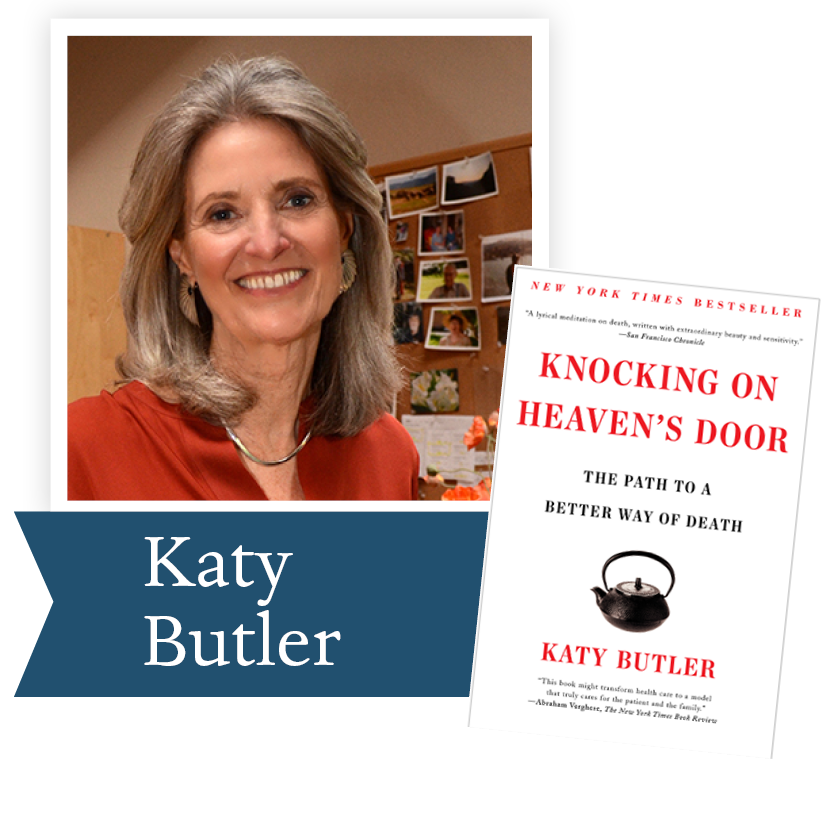Knocking on Heaven’s Door: The Path to a Better Way of Death by Katy Butler (Scribner, a division of Simon & Schuster): Pondering the medical forces that stood in the way of her own parents’ desires for “good deaths,” journalist Katy Butler examines modern medicine’s potential, in its pursuit of maximum longevity, to create more suffering than it prevents.

 table div table+table+table+table+table+table+table+table+table+table+table+table+table+table+table+table+table+table+table+table+table+table+table+table+table+table+table+table+table+table div table{width:100%;padding:0}table div table+table+table+table+table+table+table+table+table+table+table+table+table+table+table+table+table+table+table+table+table+table+table+table+table+table+table+table+table+table div table img{width:96.23%;padding:0;float:none}table div table+table+table+table+table+table+table+table+table+table+table+table+table+table+table+table+table+table+table+table+table+table+table+table+table+table+table+table+table+table div table td{width:100%;padding:0 1.88% 18px}/* styles */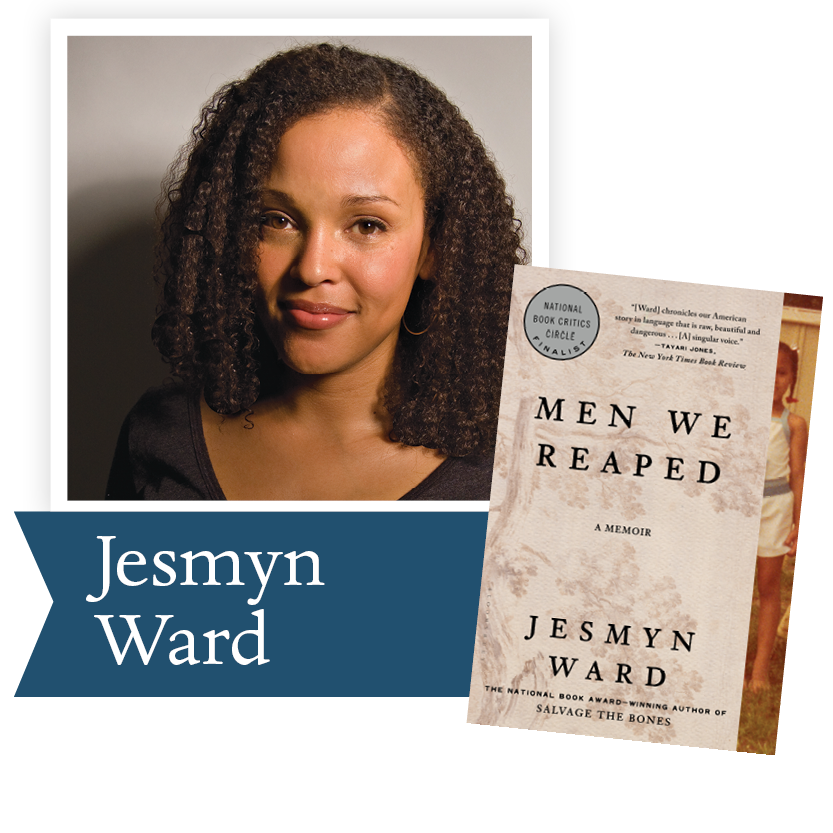Men We Reaped: A Memoir by Jesmyn Ward (Bloomsbury): In this universally acclaimed memoir, Ward recounts the separate deaths of five young men—all dear to her—from her small Mississippi community, agonizingly tracing each one back to the long-term effects of racism and economic disadvantage.

 table div table+table+table+table+table+table+table+table+table+table+table+table+table+table+table+table+table+table+table+table+table+table+table+table+table+table+table+table+table+table+table+table div table{width:100%;padding:0}table div table+table+table+table+table+table+table+table+table+table+table+table+table+table+table+table+table+table+table+table+table+table+table+table+table+table+table+table+table+table+table+table div table img{width:96.23%;padding:0;float:none}table div table+table+table+table+table+table+table+table+table+table+table+table+table+table+table+table+table+table+table+table+table+table+table+table+table+table+table+table+table+table+table+table div table td{width:100%;padding:0 1.88% 18px}/* styles */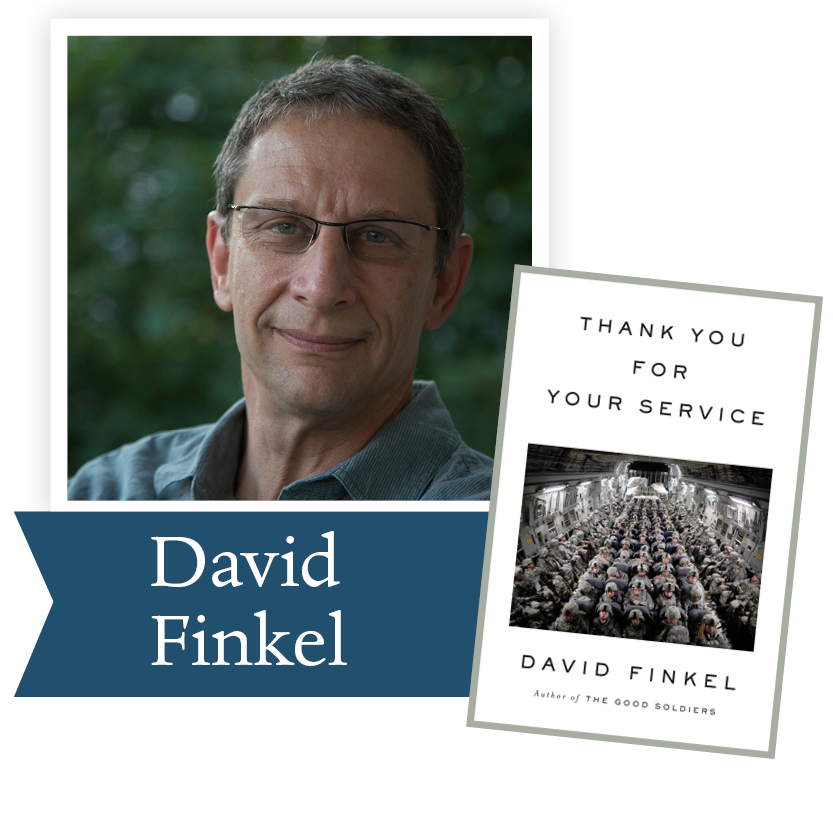Thank You for Your Service by David Finkel (Sarah Crichton Book/Farrar, Strauss and Giroux): Pulitzer Prize-winning journalist David Finkel follows veterans of the infamous Baghdad “surge” after they return to the U.S., creating an indelible, essential portrait of post-deployment life—not just
for the soldiers, but for their families, friends, and the professionals trying to undo the damage of war.

 table div table+table+table+table+table+table+table+table+table+table+table+table+table+table+table+table+table+table+table+table+table+table+table+table+table+table+table+table+table+table+table+table+table+table div table{width:100%;padding:0}table div table+table+table+table+table+table+table+table+table+table+table+table+table+table+table+table+table+table+table+table+table+table+table+table+table+table+table+table+table+table+table+table+table+table div table img{width:96.23%;padding:0;float:none}table div table+table+table+table+table+table+table+table+table+table+table+table+table+table+table+table+table+table+table+table+table+table+table+table+table+table+table+table+table+table+table+table+table+table div table td{width:100%;padding:0 1.88% 18px}/* styles */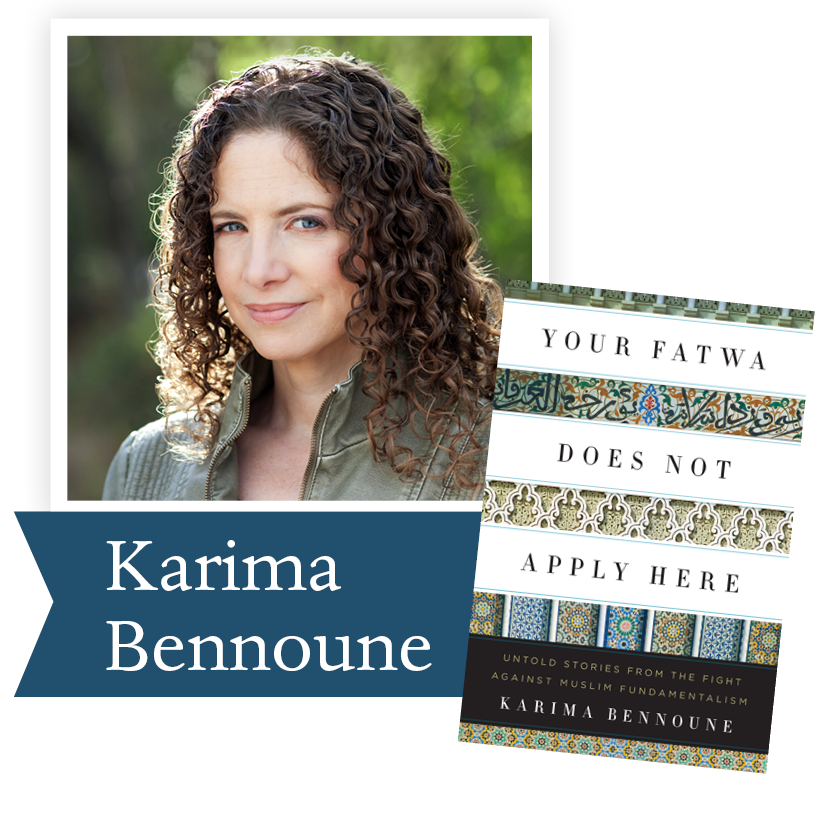Your Fatwa Does Not Apply Here: Untold Stories from the Fight Against Muslim Fundamentalism by Karima Bennoune (W.W. Norton & Company): From Karachi to Tunis, Kabul to Tehran, Bennoune shares the inspiring stories of the Muslim writers,
artists, doctors, lawyers, activists, and educators who often risk death to combat the rising tide of religious extremism in their own countries.

 table div table+table+table+table+table+table+table+table+table+table+table+table+table+table+table+table+table+table+table+table+table+table+table+table+table+table+table+table+table+table+table+table+table+table+table+table div table{width:100%;padding:0}table div table+table+table+table+table+table+table+table+table+table+table+table+table+table+table+table+table+table+table+table+table+table+table+table+table+table+table+table+table+table+table+table+table+table+table+table div table img{width:96.23%;padding:0;float:none}table div table+table+table+table+table+table+table+table+table+table+table+table+table+table+table+table+table+table+table+table+table+table+table+table+table+table+table+table+table+table+table+table+table+table+table+table div table td{width:100%;padding:0 1.88% 18px}/* styles */## 2014 Ambassador Richard C. Holbrooke Distinguished Achievement Award Winner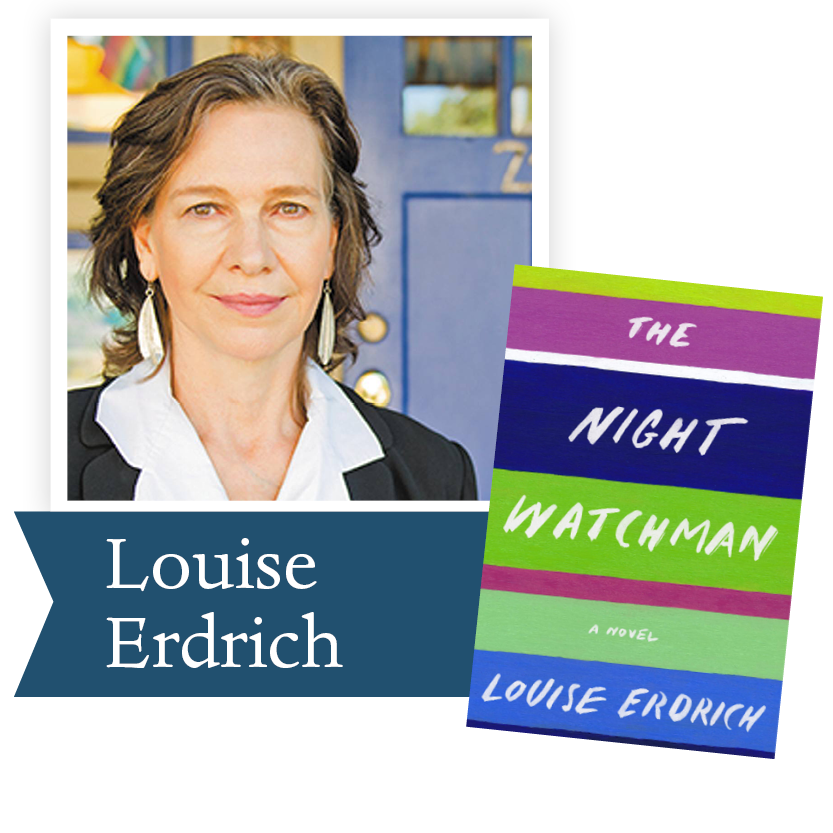We are featuring Louise Erdrich’s most recent adult novel, The Night Watchman, inspired by letters her grandfather wrote in the mid-1950’s as chairman of the Turtle Mountain Band of Chippewa Advisory Committee about their struggle against the destruction of treaties and the threat to their survival coming from Washington, DC. In this novel, as in her many others we have celebrated, Erdrich recognizes the power of community and the power of the larger sense of humanity to be the basis for all ethical action. This novel will be a future DLPP Book Club read.

We invite you to read the Washington Post review, With ‘The Night Watchman,’ Louise Erdrich rediscovers her genius.

 table div table+table+table+table+table+table+table+table+table+table+table+table+table+table+table+table+table+table+table+table+table+table+table+table+table+table+table+table+table+table+table+table+table+table+table+table+table+table+table div table{width:100%;padding:0}table div table+table+table+table+table+table+table+table+table+table+table+table+table+table+table+table+table+table+table+table+table+table+table+table+table+table+table+table+table+table+table+table+table+table+table+table+table+table+table div table img{width:96.23%;padding:0;float:none}table div table+table+table+table+table+table+table+table+table+table+table+table+table+table+table+table+table+table+table+table+table+table+table+table+table+table+table+table+table+table+table+table+table+table+table+table+table+table+table div table td{width:100%;padding:0 1.88% 18px}/* styles */table div table+table+table+table+table+table+table+table+table+table+table+table+table+table+table+table+table+table+table+table+table+table+table+table+table+table+table+table+table+table+table+table+table+table+table+table+table+table+table+table+table div table{width:100%;padding:0}table div table+table+table+table+table+table+table+table+table+table+table+table+table+table+table+table+table+table+table+table+table+table+table+table+table+table+table+table+table+table+table+table+table+table+table+table+table+table+table+table+table div table img{width:96.23%;padding:0;float:none}table div table+table+table+table+table+table+table+table+table+table+table+table+table+table+table+table+table+table+table+table+table+table+table+table+table+table+table+table+table+table+table+table+table+table+table+table+table+table+table+table+table div table td{width:100%;padding:0 1.88% 18px}/* styles */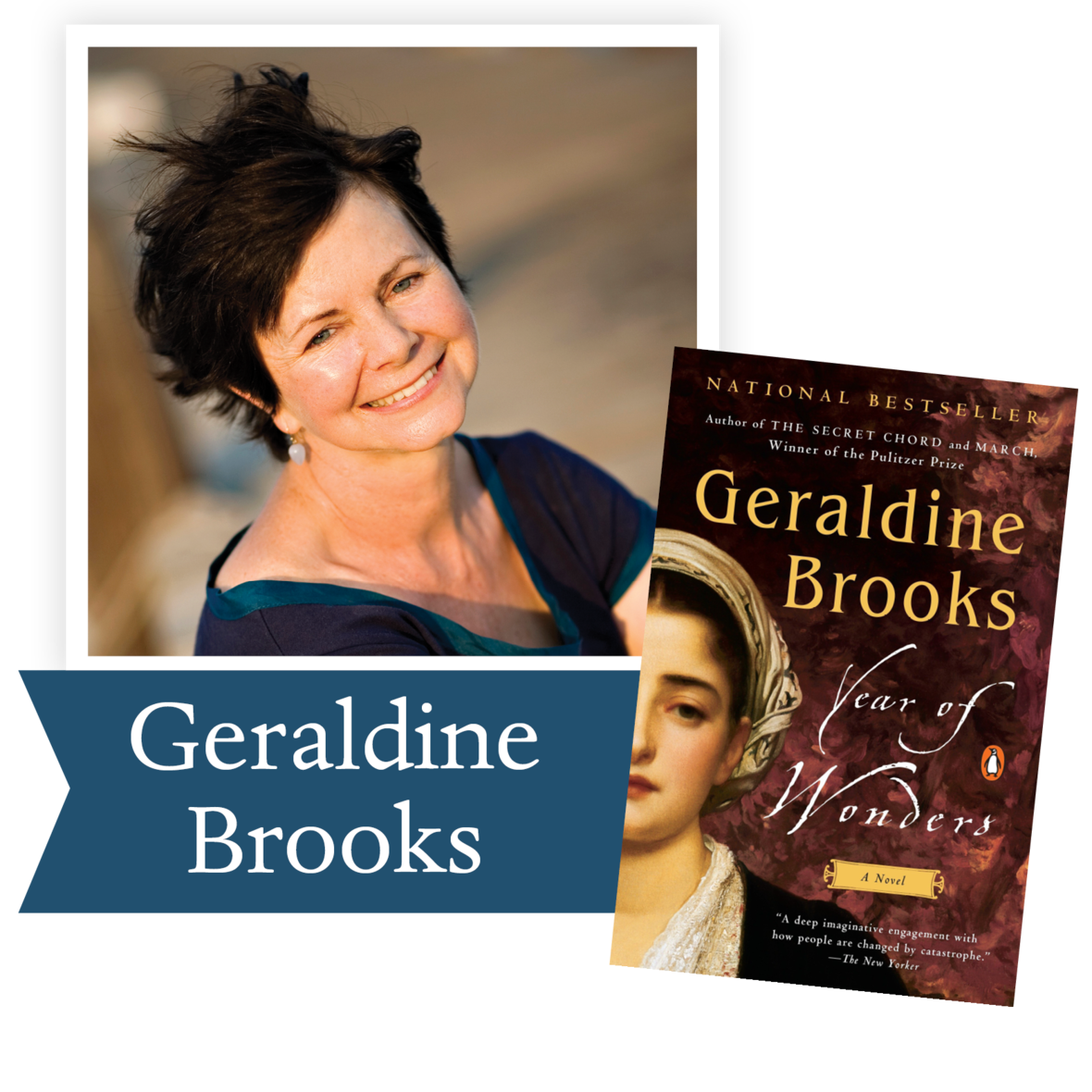“Plague stories remind us that we cannot manage without community . . . Year of Wonders is a testament to that very notion.” – The Washington Post

An unforgettable tale, set in 17th century England, of a village that quarantines itself to arrest the spread of the plague, from the author of The Secret Chord and of March, winner of the Pulitzer Prize.

Ron Rollins, Dayton Daily News Ideas and Voices Editor, will moderate the discussion of The Year of Wonders.

Join us Wednesday, May 20 at 7:30 pm via Zoom for our first book club! Email Emily Kretzer to be added to the list.

 table div table+table+table+table+table+table+table+table+table+table+table+table+table+table+table+table+table+table+table+table+table+table+table+table+table+table+table+table+table+table+table+table+table+table+table+table+table+table+table+table+table+table+table div table{width:100%;padding:0}table div table+table+table+table+table+table+table+table+table+table+table+table+table+table+table+table+table+table+table+table+table+table+table+table+table+table+table+table+table+table+table+table+table+table+table+table+table+table+table+table+table+table+table div table img{width:96.23%;padding:0;float:none}table div table+table+table+table+table+table+table+table+table+table+table+table+table+table+table+table+table+table+table+table+table+table+table+table+table+table+table+table+table+table+table+table+table+table+table+table+table+table+table+table+table+table+table div table td{width:100%;padding:0 1.88% 18px}/* styles */Support the Dayton Literary Peace Prize when you shop with Amazon using this link.